# Select

Select the statement that describes this expression: 5 x (3 + 4) - 4.

a) 5 times the sum of 3 and 4, then subtract 4
b) 5 times 3 plus 4 minus 4
c) 5 more than the sum of 3 and 4, and then subtract 4
d) 5 times 3 plus the difference of 3 and 4

What is the value of this expression (write result number)

Result

s = (Correct answer is: a)x =  31

### Step-by-step explanation:

$s=a$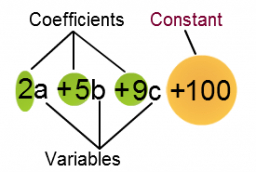Did you find an error or inaccuracy? Feel free to write us. Thank you!## Related math problems and questions:

• Expressions with variableThis is algebra. Let n represent an unknown number and write the following expressions: 1. 4 times the sum of 7 and the number x 2. 4 times 7 plus the number x 3. 7 less than the product of 4 and the number x 4. 7 times the quantity 4 more than the number
• TranslateTranslate the following mathematical statement into an algebraic expression or equation. 1. Nine less that the quotient of a number and 3 2. The ratio of 3 and the sum of 4 and an unknown number 3. Eighty is the product of 4 and g 4. The sum of x and 18 i
• Phone number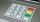Ivan's phone number ends with a four-digit number: When we subtract the first from the fourth digit of this four-digit number, we get the same number as when we subtract the second from the third digit. If we write the four-digit number from the back and
• Unknown number 5I think of an unknown number. If we enlarge it five times, then subtract 3, and the result decreases by 75%, we get one greater than the number. What number am I thinking of?
• Algebra problemThis is algebra. Let n represent an unknown number. 1. Eight more than the number n 2. Three times the number n 3. The product of the number n and eight 4. Three less than the number n 5. Three decreased by the number n
• Mysterious number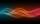The magician thinks the number: "The mysterious number is first divided by minus five, dividing the result by three, multiplying the number by ten, and dividing the resulting number by minus four. This gives result 5. Can you reveal the mysterious number?
• Even / odd numbers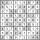a / Using variable n write two consecutive odd numbers. b / the sum of three consecutive odd numbers is 333. What are this numbers?
• Equivalent equationWhich equation, when solved, results in a different value of x than the other three? A. - (7)/(8) x - (3)/(4) = 20 Negative 7/8 x minus three-fourths = 20 B. (3)/(4) + (7)/(8) x= -20 Three-fourths + StartFraction 7 over 8 En
• Unknown number 17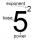Milada said, I am thinking of a number such that I evaluate expression x1/3, the value of the expression would be 5. Which number Milada thinking?
• Three numbersFind three numbers so that the second number is 4 times greater than the first and the third is lower by 5 than the second number. Their sum is 67.
• Number of songs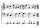Write an expression for the number of songs they need for this show. Evan and Peter have a radio show that has 2 parts. They need 4 fewer than 11 songs in the first part. In the second part, they need 5 fewer than 3 times the number of songs in the first
• Complicated sum minus productWhat must be subtracted from the sum of 3/8 and 5/16 to get difference equal to the product of 5/8 and 3/16?
• Fraction unknowns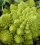Divide of fractions with unknowns: Fraction 1: The quantity x squared plus 6 times x plus 9 over the quantity x minus 1. Fraction 2: the quantity x squared minus 9 over the quantity x squared minus 2 times x plus 1.  Find Fraction 1 over Fraction 2.
• Value of expressionX=2, y=-5 and z=3 what is the value of x-2y?
• Aircraft modelsHow many car models did the collector own after the collector's market? The collector of car and aircraft models owned 3 times more car models than aircraft. He sold 7 car models and 9 aircraft models on the stock exchange and bought 12 car models and 4 a
• Two thirdsTwo thirds of the unknown number is 8/7. What is 7/3 of this number?
• A number 5A number is divisible by 24, 25, and 120 if it is increased by 20. Find the number.